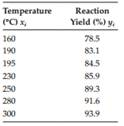Create an Account

Home / Questions / The yield of a certain chemical reaction at various temperatures is recorded in the follow...

The yield of a certain chemical reaction at various temperatures is recorded in the following table Find the reaction yield at 260°C by using a interp1 b Clamped

The yield of a certain chemical reaction at various temperatures is recorded in the following table. Find the reaction yield at 260°C by using

a. interp1.

b. Clamped (p = −1, q = 1) cubic spline interpolation. Plot both splines and the original data.

c. Clamped (p = −0.5, q = 0.5) cubic spline interpolation. Plot the new splines together with those of (a). Compare with (b) and discuss the results.Jul 22 2020 View more View LessSubscribe To Get Solution